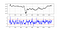# The Normalized Bollinger Indicator. Another Way to Trade the Range. Back-testing in Python.

## Digging deeper and modifying the Bollinger Bands.

First of all, what are the Bollinger Bands? This is important before we get to to the Normalized Bollinger Indicator (also known as the %B indicator). The concept is really simple, by default, the indicator calculates a 20-period simple moving average and two standard deviations away from the price, then plots them together to get a better understanding any statistical extremes. Clearly, the below chart seems easy to understand, every time the price reaches one of the bands, a contrarian position is most suited.

The %B indicator is the same as the usual Bollinger Bands, only it offers another view to detect statistical extremes from a more technical stand. The concept is also similar to the RSI’s intuition with the oversold and overbought levels. I have written about the RSI and how to combine it with the Bollinger Bands in a previous article:

Now, back to our topic. The formula to calculate the %B relies a normalization principle such as the below:

This gives us an indicator that fluctuates around some values. It will mostly remain within a range between 0 and 1.5 for most of the time (some excess will be seen). Our job now is to code a function in Python that allows us to calculate this indicator, then create a trading rule, and finally, back-test our results over a few currency pairs.

Intuitively, we can just write our Bollinger function that I have been presenting through the recent articles, and then, through it, we’ll incorporate the above function.

On an OHLC data structure, the above function will give us three new columns. The simple moving average column, the upper Bollinger band and the lower Bollinger band. Hence, to calculate the %B indicator, we will need the fourth column referring to the closing price (Data[:, 3]), the sixth column referring to the upper band (Data[:, 5]), and the seventh column referring to the lower band (Data[:, 6]). This gives us a simple line of code that calculates the indicator:

Let’s now chart it to see what it looks likeGBPUSD H3 Time Frame in the first panel with %B in the second panel.

Our signals will be simply to buy whenever the indicator hits -0.4 and to sell (go short) whenever it hits 1.4. The below function details more the signals:

To quickly explain the function before we move on; It will loop through the dataset, look for the indicator’s values (represented by the boll_percentage variable) and check whether they are less than the lower barrier or greater than the upper barrier. Now, to show the signals chart on the GBPUSD:

Notice that the indicator sometimes picks up the tops and bottoms with a good timing potential. Recently with prices trending upwards, it has been less effective in doing so but the below equity curve shows that it can add value over the long-term. Of course, this does not mean that it should be used as a strategy. Note that the holding period for the strategy was 20 periods (20 x 3 = 60 hours).

With a 48.14% hit ratio and a net profit of \$4443.8, this strategy does seem to provide some value albeit the under 50% hit ratio. The realized risk-ratio is 1.24 and the expectancy of each trade is \$7.17. Finally, the Sharpe ratio is at 0.05 after 619 trades. To understand more these performance metrics, you can refer to a previous article:

# Conclusion

It can be interesting to try it out on the other pairs through different time frames and by optimizing the %B parameters such as the barriers and the lookback period. Risk management tools must be added as well to make this strategy tradeable.